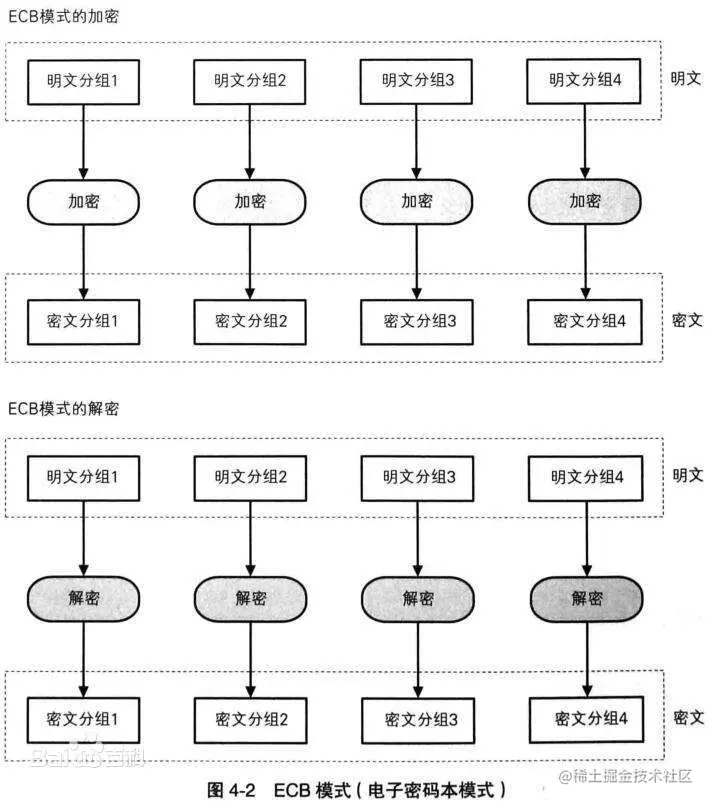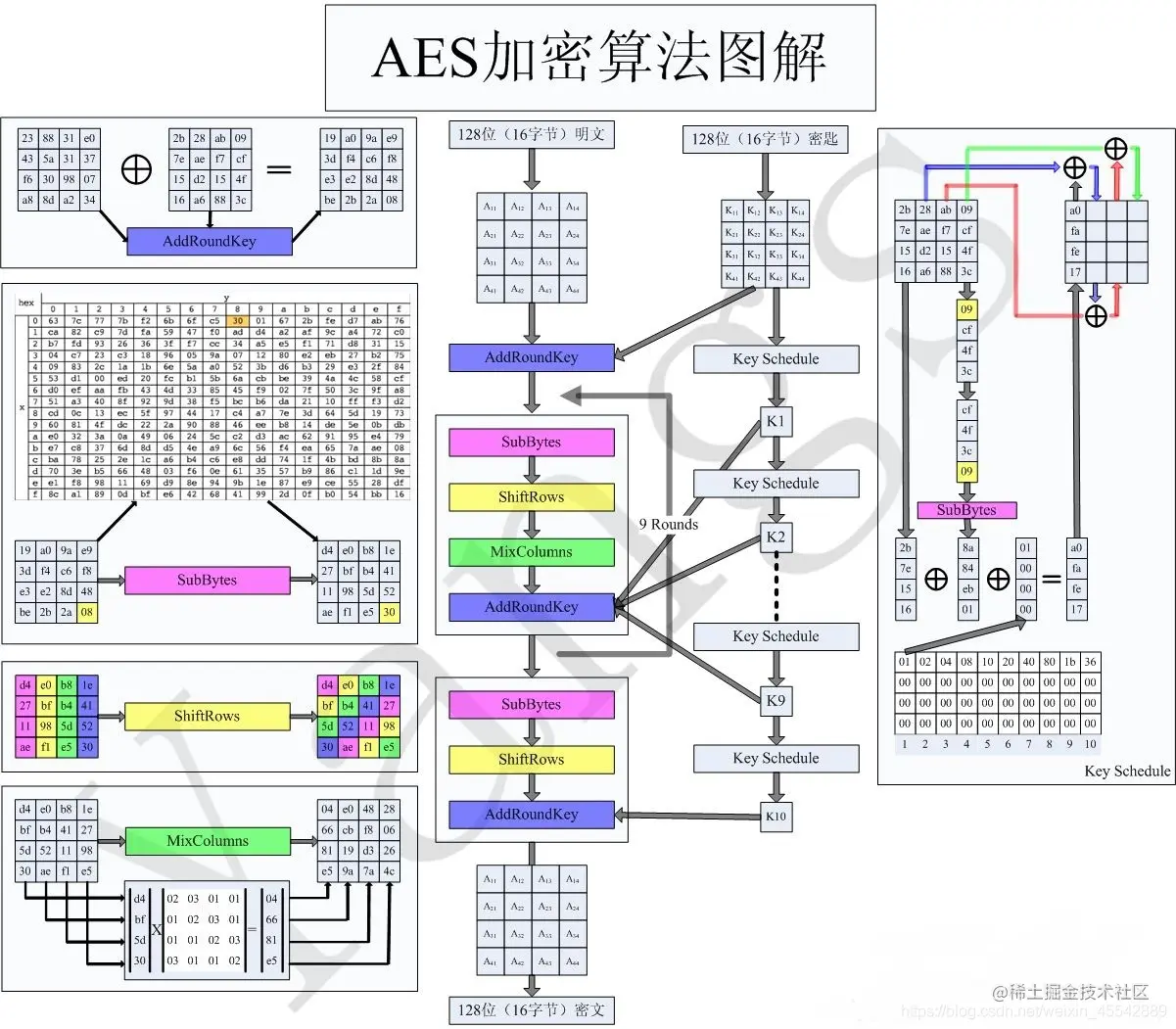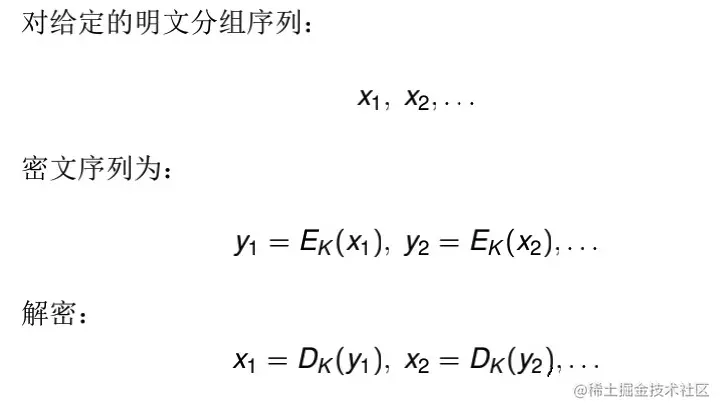# Java AES / ECB / pkcs5padding encryption conversion Python 3

2022-01-31 02:43:49 qizai

## java AES/ECB/PKCS5padding encryption transformation Python

Be careful ： encryption 128 position AES The encryption mode is ECB To encrypt , Use after encryption Base64 transcoding .

Recently with java Project docking encountered AES encryption algorithm ,java The code has SecureRandom.getInstance("SHA1PRNG"); convert to Python## AES encryption`````` Advanced encryption standard （Advanced Encryption Standard: AES） National Institute of standards and technology （NIST） stay 2001 The encryption specification of electronic data was established in .
It is one of the most classical algorithms of symmetric encryption and decryption algorithm , It is a packet encryption standard , The size of each encryption block is 128 position , The allowed key length is 128、192 and 256 position . This is just an introduction ECB Encryption mode .

AES Encryption mode ：ECB/CBC/CTR/OFB/CFB
Data blocks ：128 position /192 position /256 position
Copy code ``````

## ECB Pattern

ECB Mode as the simplest working mode , Group plaintext directly , Each group is encrypted separately , Each group is independent and irrelevant## Java Default encryption demo

Java Default AES The encryption mode is "AES/ECB/PKCS5Padding".

java Code display

``````package com.qzd.hit.util;

import javax.crypto.Cipher;
import javax.crypto.SecretKey;
import javax.crypto.spec.SecretKeySpec;

public class Demo {

/*** main * @author qizai * data: params * encStr: Here, the length of the key must be a multiple of 16 */
public static void main(String[] args) throws Exception {
// Encrypt Data
String key = "ROcb6JybnKILLOlO"; // Secret Key length must be (16 24 32)
String data = "[\"123456789\"]"; // Content to be encrypted
String encStr = encrypt(data, key);  // Content to be decrypted
System.out.println(encStr); //Encrypted data: w6oijM0xddQrItnH3UybLQ==
// Decrypt Data
String decStr = decrypt(encStr, key);
System.out.println(decStr); //Decrypted data: ["123456789"]
}

public static String encrypt(String content, String password) {
if (StringUtils.isEmpty(content) || StringUtils.isEmpty(password)) {
System.out.println("AES encryption params is null");
return null;
}
try {
// Create cipher
Cipher cipher = Cipher.getInstance("AES/ECB/PKCS5Padding");
byte[] byteContent = content.getBytes("UTF-8");
// Initialize as cryptographic cipher
byte[] encryptByte = cipher.doFinal(byteContent);
return org.apache.commons.codec.binary.Base64.encodeBase64String(encryptByte);
} catch (Exception e) {
System.out.println("AES encryption operation has exception,content:{},password:{}", content, password, e);
}
return null;
}

public static String decrypt(String encryptContent, String password) throws Exception {
if (StringUtils.isEmpty(encryptContent) || StringUtils.isEmpty(password)) {
System.out.println("AES The request parameter is null");
return null;
}
Cipher cipher = null;
// Set to decryption mode
// Perform decryption operation
byte[] result = cipher.doFinal(org.apache.commons.codec.binary.Base64.decodeBase64(encryptContent));
return new String(result, "UTF-8");
}

private static SecretKeySpec getSecretKey(final String password) throws NoSuchAlgorithmException {
// Generates the generator for the specified algorithm key
KeyGenerator keyGenerator = KeyGenerator.getInstance("AES");
SecureRandom random = SecureRandom.getInstance("SHA1PRNG");
keyGenerator.init(128, random);
// Grnerate Key
SecretKey secretKey = keyGenerator.generateKey();
// Key converted to AES
return new SecretKeySpec(secretKey.getEncoded(), "AES");
}
}
Copy code ``````

Encryption precautions :

``````KeyGenerator keyGenerator = KeyGenerator.getInstance("AES"); // Generates the generator for the specified algorithm key
SecureRandom random = SecureRandom.getInstance("SHA1PRNG"); // SHA1PRNG Random number algorithm
keyGenerator.init(128, random);
SecretKey secretKey = keyGenerator.generateKey();
Copy code ``````

## Python Realization AES/ECB/PKCS5padding

install AES Correlation Library (window Next )

``````pip install pycryptodome
Copy code ``````

Linux Lower installation

``````pip install pycrypto
Copy code ``````

Relevant code implementation steps

``````import base64
import hashlib

import requests
from Crypto.Cipher import AES as _AES

class AES:

def __init__(self, key: str):
"""Init aes object used by encrypt or decrypt. AES/ECB/PKCS5Padding same as aes in java default. """

self.aes = _AES.new(self.get_sha1prng_key(key), _AES.MODE_ECB)

@staticmethod
def get_sha1prng_key(key: str) -> bytes:
"""encrypt key with SHA1PRNG. same as java AES crypto key generator SHA1PRNG. SecureRandom secureRandom = SecureRandom.getInstance("SHA1PRNG" ); secureRandom.setSeed(decryptKey.getBytes()); keygen.init(128, secureRandom); :param string key: original key. :return bytes: encrypt key with SHA1PRNG, 128 bits or 16 long bytes. """

signature: bytes = hashlib.sha1(key.encode()).digest()
signature: bytes = hashlib.sha1(signature).digest()
return signature[:16]

@staticmethod
def padding(s: str) -> str:

pad_num: int = 16 - len(s) % 16

@staticmethod

padding_num: int = ord(s[-1])

def encrypt_to_bytes(self, content_str):
"""From string encrypt to bytes ciphertext. """

ciphertext_bytes = self.aes.encrypt(content_bytes)
return ciphertext_bytes

def encrypt_to_base64(self, content_str):
"""From string encrypt to base64 ciphertext. """

ciphertext_bytes = self.encrypt_to_bytes(content_str)
ciphertext_bs64 = base64.b64encode(ciphertext_bytes).decode()
return ciphertext_bs64

def decrypt_from_bytes(self, ciphertext_bytes):
"""From bytes ciphertext decrypt to string. """

content_bytes = self.aes.decrypt(ciphertext_bytes)
return content_str

def decrypt_from_base64(self, ciphertext_bs64):
"""From base64 ciphertext decrypt to string. """

ciphertext_bytes = base64.b64decode(ciphertext_bs64)
content_str = self.decrypt_from_bytes(ciphertext_bytes)
return content_str

def encrypt_to_bytes(content_str, encrypt_key: str):
"""From string encrypt to bytes ciphertext. """

aes: AES = AES(encrypt_key)
ciphertext_bytes = aes.encrypt_to_bytes(content_str)
return ciphertext_bytes

def encrypt_to_base64(content_str, encrypt_key: str) -> str:
"""From string encrypt to base64 ciphertext. """

aes: AES = AES(encrypt_key)
ciphertext_bs64 = aes.encrypt_to_base64(content_str)
return ciphertext_bs64

def decrypt_from_bytes(ciphertext_bytes, decrypt_key: str) -> str:
"""From bytes ciphertext decrypt to string. """

aes: AES = AES(decrypt_key)
content_str = aes.decrypt_from_bytes(ciphertext_bytes)
return content_str

def decrypt_from_base64(ciphertext_bs64, decrypt_key: str) -> str:
"""From base64 ciphertext decrypt to string. """

aes: AES = AES(decrypt_key)
content_str = aes.decrypt_from_base64(ciphertext_bs64)
return content_str

if __name__ == "__main__":
key = "iC6qfFyiza8aq"
encrypt_str_data = "[\"13811111111\"]"
et = encrypt_to_base64(encrypt_str_data, key)
print(" Encrypted data : ", et)
ret = decrypt_from_base64(et, key)
print(" Decrypted data : ", ret)

Copy code ``````

Key code

This method realizes the right of key Transformation ,java Content of key encryption code in , Return here as 16 Base string .

`````` @staticmethod
def get_sha1prng_key(key: str) -> bytes:
"""encrypt key with SHA1PRNG. same as java AES crypto key generator SHA1PRNG. SecureRandom secureRandom = SecureRandom.getInstance("SHA1PRNG" ); secureRandom.setSeed(decryptKey.getBytes()); keygen.init(128, secureRandom); :param string key: original key. :return bytes: encrypt key with SHA1PRNG, 128 bits or 16 long bytes. """

signature: bytes = hashlib.sha1(key.encode()).digest()
signature: bytes = hashlib.sha1(signature).digest()
return signature[:16]
Copy code ``````

## Simple version

``````import base64
import hashlib

from Crypto.Cipher import AES

SECRET_KEY = 'iC6qfFyiza8aq'  #  secret key

class AesEncrypt(object):
def __init__(self):
self.key = self.getKey(SECRET_KEY)
self.mode = AES.MODE_ECB

""" Processing of encrypted characters """
return text + (len(self.key) - len(text) % len(self.key)) * chr(len(self.key) - len(text) % len(self.key))

""" Processing of decrypted characters """
return text[0:-ord(text[-1:])]

def getKey(self, key):
""" Yes key To deal with ,key  The length of  16,24,32"""
signature: bytes = hashlib.sha1(key.encode()).digest()
signature: bytes = hashlib.sha1(signature).digest()
return signature[:16]

def encrypt(self, text):
""" Encryption function """
ecb_encrypt = AES.new(self.key, self.mode)  # ECB  Pattern
ecb_text = ecb_encrypt.encrypt(bytes(self.pading(text), encoding="utf8"))
encrypt_string = base64.b64encode(ecb_text).decode()
return encrypt_string

def decrypt(self, text):
""" Decryption function """
decode = base64.b64decode(text)
ecb_decrypt = AES.new(self.key, self.mode)  # ECB  Pattern
plain_text = ecb_decrypt.decrypt(decode)
return decrypt_string

if __name__ == '__main__':
aes_encrypt = AesEncrypt()
data = "[\"13811111111\"]"
en = aes_encrypt.encrypt(data)
print(" Encrypt data : ", en)
dr = aes_encrypt.decrypt(en)
print(" Decrypt data : ", dr)

Copy code ``````

1. Yes key Conduct sha1prng encryption , It's in the text get_sha1prng_key Method , Get the encrypted ciphertext as AES Of key

2. determine AES Encryption method of ECB,CBC etc.  AES Encryption mode ：ECB/CBC/CTR/OFB/CFB

3. fill ,key And the original text can be filled ,NoPadding, No fill ,0 fill , also pkcs5padding, Not filling is not filling the content , Direct encryption , The code above implements \0 Filling and pkcs5padding .

4. Generally speaking, encrypted content is basically key The processing is different or the filling is wrong .

【 Reference resources SHA1 Algorithm 】